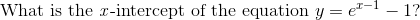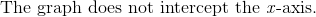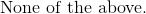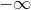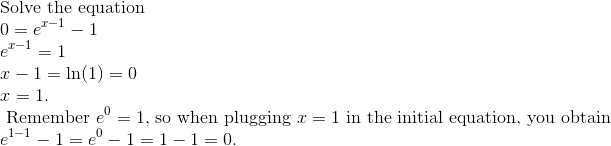# Algebra 1 : How to graph an exponential function

## Example Questions

### Example Question #1 : How To Graph An Exponential Function

What is the horizontal asymptote of the graph of the equation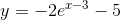?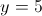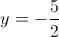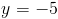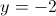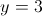Explanation:

The asymptote of this equation can be found by observing that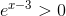regardless of. We are thus solving for the value ofas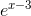approaches zero.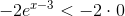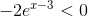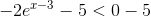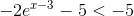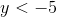So the value thatcannot exceed is, and the line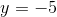is the asymptote.

### Example Question #641 : Exponents

What is/are the asymptote(s) of the graph of the function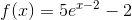?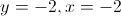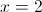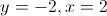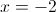Explanation:

An exponential equation of the form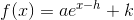has only one asymptote - a horizontal one at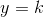. In the given function,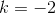, so its one and only asymptote is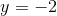.

### Example Question #1 : How To Graph An Exponential Function